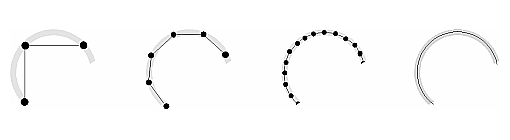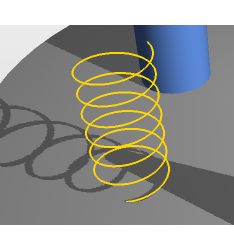Line Segments

# Introduction

In geometry, a line segment is a part of a line that is bounded by two distinct end points, and contains every point on the line between its end points. A closed line segment includes both endpoints.Line segments can be used to approximate smooth curves.

# Line Segment ObjectsCreating a spring using an list of segments. See example 13-primitives

Similar to mesh objects, line segment models use vertices to describe all the lines that compose the objects. A finger-proxy algorithm is also used to compute all the interaction forces between the haptic tool and the object. A collision detection must also be initialized after all points have been created.

using namespace chai3d;
// create a line segment object
cMultiSegment* segments = new cMultiSegment();
// add object to world
// connect some segments to form a spring
double h = 0.0;
double dh = 0.001;
double a = 0.0;
double da = 0.2;
double r = 0.05;
for (int i=0; i<200; i++)
{
double px0 = r * cos(a);
double py0 = r * sin(a);
double pz0 = h;
double px1 = r * cos(a+da);
double py1 = r * sin(a+da);
double pz1 = h+dh;
// create vertex 0
int index0 = segments->newVertex(px0, py0, pz0);
// create vertex 1
int index1 = segments->newVertex(px1, py1, pz1);
// create segment by connecting both vertices together
segments->newSegment(index0, index1);
h = h + dh;
a = a + da;
}
// set haptic properties
segments->m_material->setStiffness(0.5 * maxStiffness);
// assign color properties
cColorf color;
color.setYellowGold();
segments->setLineColor(color);
// assign line width
segments->setLineWidth(4.0);
// use display list for faster rendering
segments->setUseDisplayList(true);
// build collision tree